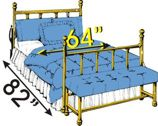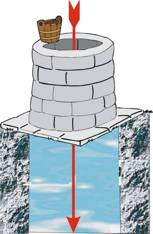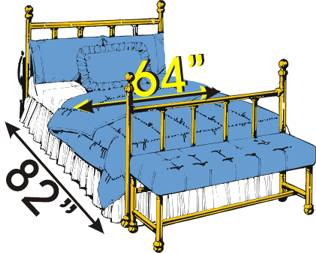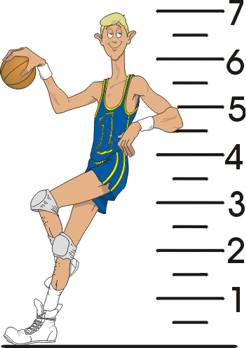# Lesson 31 - How + Adjectives - Exercise 12

### Another use of "How long..." (How + Adjectives)

How wide - How deep - How long

"How long" is also used with the verbs "is" and "are" to know the length of something. Look at the examples:
Example: How long is the pipeline?How wide is the bed? = What is the width of the bed?How deep...? = What is the depth of...?How big...? = What is the size of...?
How tall...? = What is the height of...?
How far...? = What is the distance...?
How high...? = What is the height of...?

```How do we measure length? In the USA, we still use inches, feet, yards, and miles:
1 inch = 2.54 cm
1 foot = 30.48 cm
1 yard = 914 cm
1 mile = 1.6 km```

Question: How wide is the bed?
Answer: The bed is 64 inches wide.Look at the picture. Read and listen. Type the question. Then click on "Check".
Tall:Answer: He is 6 feet 11 inches.

Type the question:

Note: When we speak about objects, buildings, for example, we can ask "How high is it?", but it is not possible to ask "how high" a person is when speaking about dimensions. We use a a different adjective beginning with a "t"!
Tap on any word once (mobile), or double-click on any word (computer), to read an English definition. If you need an approximate translation to your own language, the Google Translate button is available at the top of the screen.